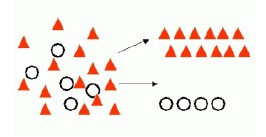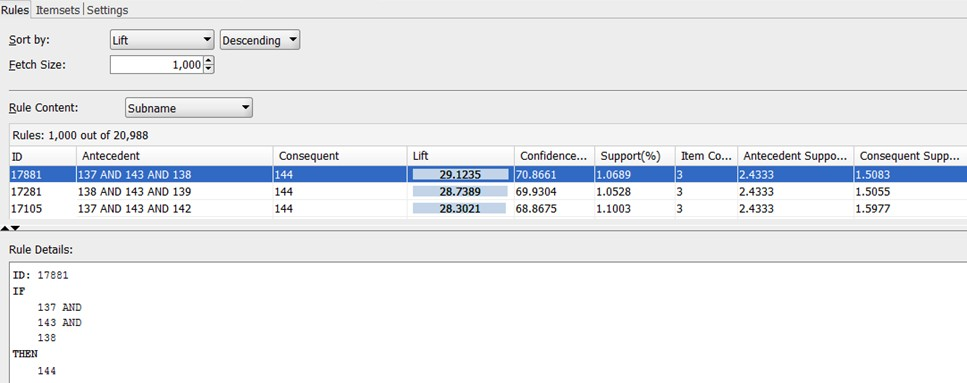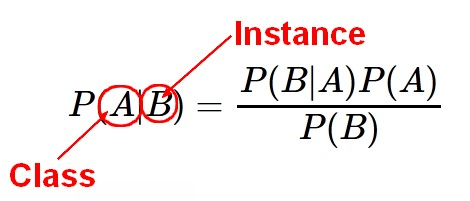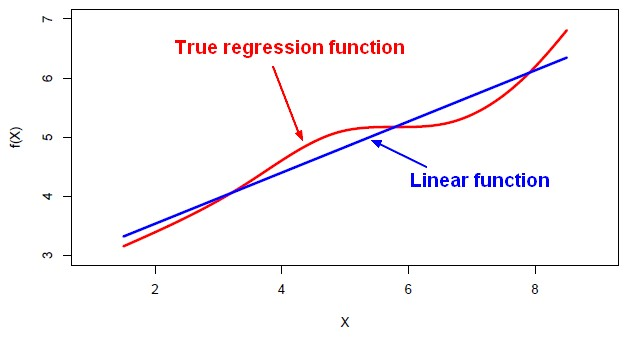# Machine Learning - (One|Simple) Rule - (One Level Decision Tree)

One Rule is an simple method based on a 1‐level decision tree described in 1993 by Rob Holte, Alberta, Canada.

Simple rules often outperformed far more complex methods because some datasets are :

• really simple
• so small/noisy/complex that nothing can be learned from them

## Implementation

### Basic

• One branch for each value
• Each branch assigns most frequent class
• Error rate: proportion of instances that don’t belong to the majority class of their corresponding branch
• Choose attribute with smallest error rate
``````For each attribute,
For each value of the attribute,
make a rule as follows:
count how often each class appears
find the most frequent class
make the rule assign that class to this attribute-value
Calculate the error rate of this attribute’s rules
Choose the attribute with the smallest error rate```
```

Example of output for the weather data set

``````outlook:
if sunny	-> no
if overcast	-> yes
if rainy	-> yes
```
```

with this one-level decision tree, 10 instances are correct on 14.

### Other

Algorithm to choose the best rule

``````For each attribute:
For each value of that attribute, create a rule:
1. count how often each class appears
2. find the most frequent class, c
3. make a rule "if attribute=value then class=c"
Calculate the error rate of this rule
Pick the attribute whose rules produce the lowest error rate
```
```

## One Rule vs Baseline

OneR always outperforms (or, at worst, equals) Baseline when evaluated on the training data. (evaluating on the training data doesn't reflect performance on independent test data.)

ZeroR sometimes outperforms OneR if the target distribution is skewed or limited data is available, predicting the majority class can yield better results than basing a rule on a single attribute. This happens with the nominal weather dataset

## minBucket Size

The “minBucket size” parameter of weka limits the complexity of rules in order to avoid overfitting (Default 6)

With one “minBucket size” the accuracy on the training data set is really high and decreases whereas the “minBucket size parameter” increases.

The cross validation evaluation method (10 folders) limits the accuracy effect and make it more stable through the “minBucket size” values.

min
Bucket
Size
Parameter
Eval
Method:
Cross
Valid-
ation
Accuracy
Eval
Method:
Training
Set
Accuracy
Number
of
conditions
generated
1 47.66 92.99 106
2 48.13 71.5 31
3 59.81 68.22 14
4 59.35 66.36 10
5 57.94 63.55 8
6 57.94 63.08 8
7 58.41 62.14 6
8 56.07 61.68 6
9 57.48 60.75 4
10 57.94 59.34 4

Recommended PagesData Mining - (Classifier|Classification Function)

A classifier is a Supervised function (machine learning tool) where the learned (target) attribute is categorical (“nominal”) in order to classify. It is used after the learning process to classify...Data Mining - (Decision) Rule

Some forms of predictive data mining generate rules that are conditions that imply a given outcome. Rules are if-then-else expressions; they explain the decisions that lead to the prediction. They...Data Mining - Decision Tree (DT) Algorithm

Desicion Tree (DT) are supervised Classification algorithms. They are: easy to interpret (due to the tree structure) a boolean function (If each decision is binary ie false or true) Decision trees...Data Mining - Naive Bayes (NB)

Naive Bayes (NB) is a simple supervised function and is special form of discriminant analysis. It's a generative model and therefore returns probabilities. It's the opposite classification strategy...Machine Learning - Decision Stump

It makes a binary split on one of the attributes. It's considered as weak learner“ because it can only produce a tree with one level as One Rule. The boosting algorithm AdaBoostM1 utilizes it by default...Machine Learning - Linear (Regression|Model)

Linear regression is a regression method (ie mathematical technique for predicting numeric outcome) based on the resolution of linear equation. This is a classical statistical method dating back more...Statistics - (Confidence|likelihood) (Prediction probabilities|Probability classification)

Prediction probabilities are also known as: confidence (How confident can I be of this prediction?). or likelihood: (How likely is this prediction to be true?) They gives the probability of a predicted...